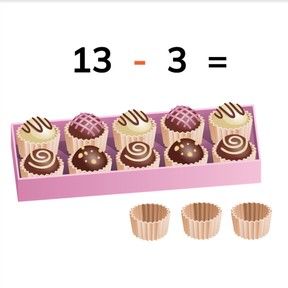Subtracting tens and ones from numbers to 20

# Subtracting tens and ones from numbers to 208,000 schools use Gynzy92,000 teachers use Gynzy1,600,000 students use Gynzy

## General

Students learn to subtract numbers to 20 with tens.

1.OA.C

## Relevance

Understanding that numbers can be split into their ten and ones makes subtraction easier and faster. An example is if you have a box with 10 chocolates and a few loose chocolates, you can quickly see how many have been eaten and how many are left.

## Introduction

Start the lesson by completing the number sequences. By doing so you refresh counting forward and backward to 20. Then do a counting exercise, in which you count chocolates. Ask students how they counted. Students might mention that it is easier to first count the sent of 10 as one set and then count on.

## Development

Show students what tens and ones are by using the box of 10 chocolates and the loose chocolates. Tell students that when they are subtracting with numbers between 10 and 20 that when you take away the ones, you are left with a ten. Demonstrate this using the subtraction problem 13 - 3 = 10 represented by the chocolates. Do three more of these problems with the students. Then tell students that when they subtract with numbers between 10 and 20 and they subtract the ten that there are ones left. Demonstrate this with the subtraciton problem 13 - 10 = 3 represented by the chocolates. Do more of these subtraction problems with the students.
Repeat the definition of tens and ones to the students, but use the blocks on the interactive whiteboard. Use these to form a subtraction problem. Then solve, using the blocks as reference. Show students that they can take away the ones and are left with ten, or that they can take away the ten and are left with ones. You can also show this with actual blocks at the front of the class. Then show the students the fill in the blank subtraction problems. Tell students that either the ten or ones are missing from the problem and must be filled in. Tell students that they must look at the difference to determine which number correctly fills in the blank. Check their understanding of these subtraction problems by doing a few as a class. Then explain to students that you can also do these subtraction problems without visual support, but by imagining the blocks in their head. Do a few subtraction problems without visual support as a class.

Check that students are able to subtract numbers to 20 in tens by asking the following questions:
- How many ones are in 14?
- How many blocks are in a ten?
- What is 16 minus 6?
- What is 16 minus 10?

## Guided practice

Students are first given subtraction problems with visual support, first subtracting ones, and then subtracting ten. Students must then choose from multiple choice answers for a subtraction problem without visual support.

## Closing

Repeat with students what tens and ones are. Ask why it is important to be able to subtract with tens. It makes calculation easier and faster. To close, do some subtraction problems with the students. First they are asked to determine the amount of ones required, then the ten, then the difference and finally are asked to solve the entire subtraction problem. They start with subtraction problems with visual support and close with subtraction problems without visual support.

## Teaching tips

Students who have difficulty with this learning goal can be supported by expanding on their knowledge of tens (group of 10) and ones (numbers to 9). They can use MAB blocks with a group of 10 and loose blocks. Remind students that they don't need to count the 10 blocks that make up the group of 10 because they already know that it is ten. This increases ease and speed of calculation.

## Instruction materials

optional- MAB blocks

### The online teaching platform for interactive whiteboards and displays in schools

• Save time building lessons

• Manage the classroom more efficiently

• Increase student engagement